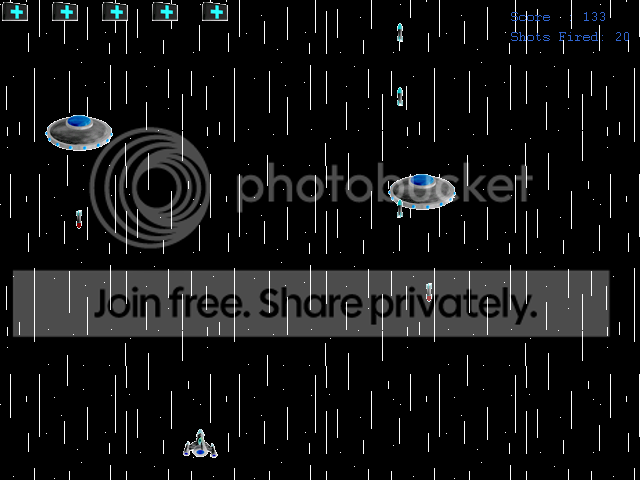-=+=- -=+=- -=+=- -=+=- -=+=- -=+=- -=+=- -=+=- -=+=- -=+=- -=+=- -=+=- -=+=- -=+=- -=+=- -=+=- -=+=- -=+=- -=+=- -=+=- -=+=- -=+=- -=+=- -=+=- -=+=- -=+=- -=+=- -=+=- -=+=- -=+=- (c) WidthPadding Industries 1987 0|563|0 -=+=- -=+=- -=+=- -=+=- -=+=- -=+=- -=+=- -=+=- -=+=- -=+=- -=+=- -=+=- -=+=- -=+=- -=+=- -=+=- -=+=- -=+=- -=+=- -=+=- -=+=- -=+=- -=+=- -=+=- -=+=- -=+=- -=+=- -=+=- -=+=- -=+=-### lyons

View Profile, Send Private Message
Recent Posts, Showcases, Articles, Code Snippets, Links, Wed.Workshop, Blog

### Latest Blog Entries

lyons23rd September 2008

Ok so i finaly decided to jump from jave to c.
but rather than programming for the computer i decided i would go for psp. mainly because i don't play it much these days, just use it for music videos and stuff.

Anyway after about 5 hours of installing t*snip*

lyons# christmas for me

27th December 2007

now that Christmas is over i decided to post a blog of what my Christmas was like.

it didn't quite feel like Christmas to me this year, i guess all the magic is really only felt by kids these days.
it just seems like an excuse to drunk (and trust me th*snip*

lyons# stop coding in blitz for a while

3rd December 2007

ok i will not be coding in blitz for a few weeks becouse my dell charger for the laptop i code on is broken.i could fix it but i need a new solder to do so,
it will be while though sence moneys tight for me at the the minute.

i might start coding on *snip*

lyons# don't know how to delete

1st October 2007

hay im lyons, being coding with blitz basic and blitz 3d for the last 5 years, moving on to java and c++ now...

### Latest Showcases

lyonsa crappy GPFT chapter 13 major edit.